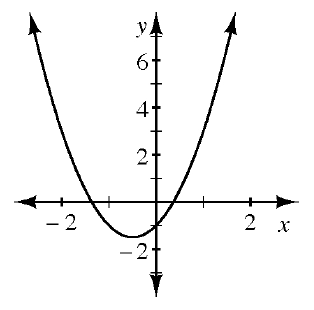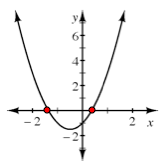### Home > INT2 > Chapter 5 > Lesson 5.2.2 > Problem5-79

5-79.Examine the graph of $y = 2x^{2} + 2x - 1$ at right.

1. Estimate the $x$-intercepts of the parabola.$x$-intercepts $\approx−1.4$ and $0.4$

2. What happens if you try to use the Zero Product Property to determine the zeros of $2x^2 + 2x - 1 = 0$?